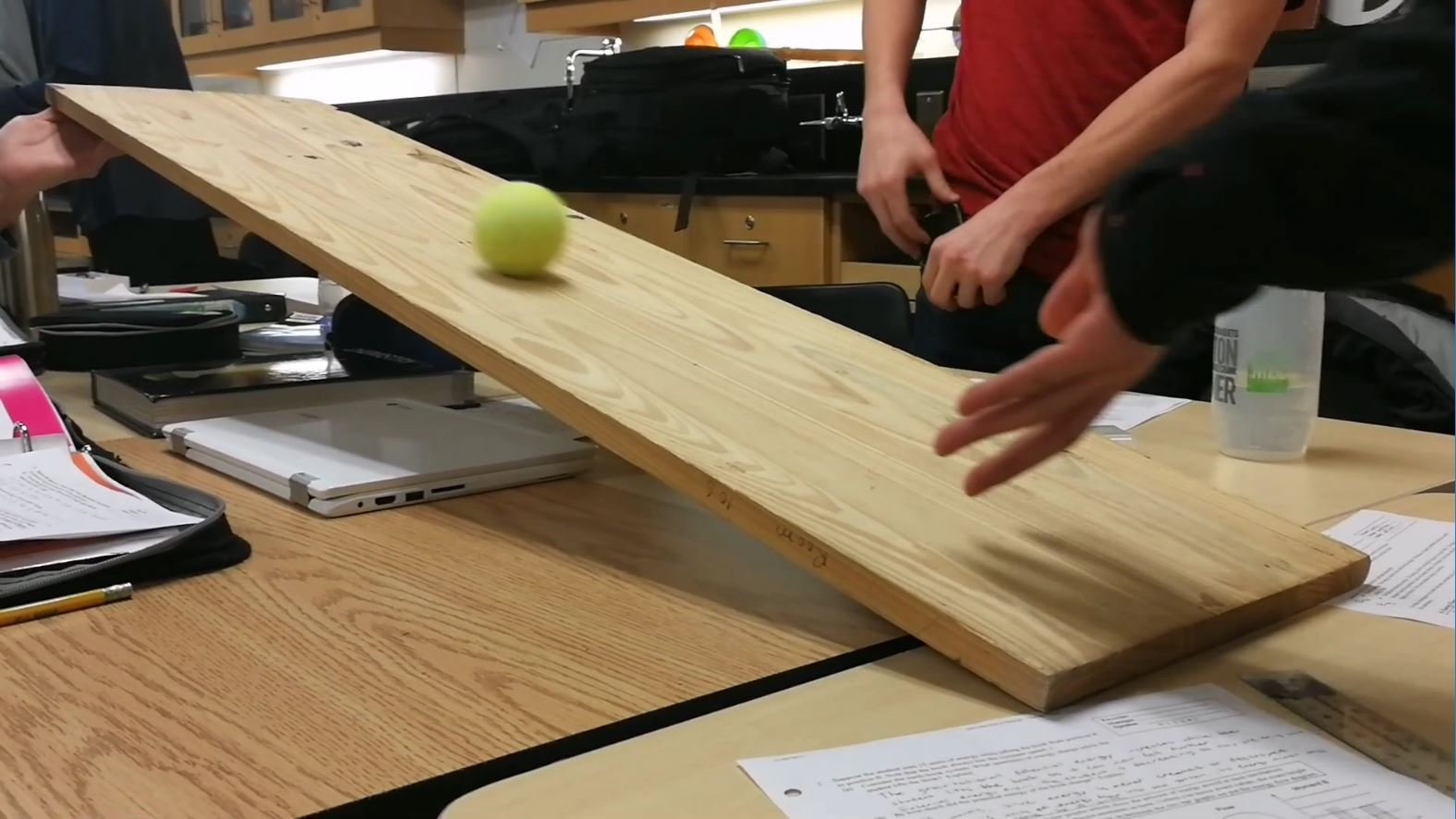## Introducing Energy!

Today, we scratched the surface of how energy flows in our world.

From our group investigation on energy, I learned an incredible amount about energy! Our group investigation involved throwing a tennis ball up a ramp and observing the flow of energy. We didn’t completely finish our investigation, but here’s what I’ve learned so far:

1) Energy is a measure of a system’s ability to do work. Work is the measure of force exerted for a certain distance. To calculate work, we use the formula Work = Force x Displacement. Both work and energy are measured in joules, named after James Prescott Joule who discovered the law of conservation of energy.

2) The law of conservation of energy states that energy can never be created or destroyed, only moved around and transformed. Two forms of energy include kinetic energy and gravitational potential energy. Kinetic energy is energy stored in the motion of an object. Gravitational potential energy is the energy stored in an object from its potential to fall down and convert itself into kinetic energy. Heavier and higher objects have more gravitational potential energy.

Note in the video above how the initial kinetic energy from throwing the ball is transformed into gravitational potential energy. Once the ball runs out of kinetic energy, the gravitational potential energy transforms back into kinetic energy, causing it to roll back down.

3) We use energy flow diagrams, which are drawn in the shape of an LOL, to depict how energy changes between two moments in time. The two Ls of the LOL diagram are bar graphs of each type of energy we want to show. The O of the diagram is a schema of the energy system; we draw the objects within and outside of the circle to depict which objects are part of the system and then draw arrows to indicate the flow of energy.

I still have questions about how energy works.

1) How do we actually calculate the gravitational potential energy or kinetic energy of an object? What can we do with our understanding of energy?

2) What are some of the other types of energy? How do we calculate those?

Something very intriguing is how crazy the derived SI units can get. With energy and work, we use joules, which are kilogram metres squared per second squared: kgm2s-2.

We can get more crazy than that. Power is measured in watts; a watt is a joule divided by a second: kgm2s-3. Electrical potential energy is measured in volts; a volt is a watt divided by an ampere: kgm2s-3A-1. Resistance is measured in ohms; an ohm is a volt divided by an ampere: kgm2s-3A-2, and so we get one of the most crazy SI units expressed in base SI units. Imagine having to say “kilograms metres squared per second cubed per ampere squared” just to express the resistance of an electrical conductor!

#### After Unit Reflection

##### Investigation of Energy Transformations

In this blog post, I did a brief investigation into the law of conservation of energy and energy transformations between mechanical forms of energy. I learned about the law of conservation of energy, which states that energy can never be created or destroyed, only moved around and transformed. I also learned how energy can be transformed from one type to another, specifically how kinetic energy transforms into gravitational potential energy (and vice versa). Kinetic energy transforms into gravitational potential energy by moving the object upwards. This increased height gives the object the potential to fall back down and gain kinetic energy.